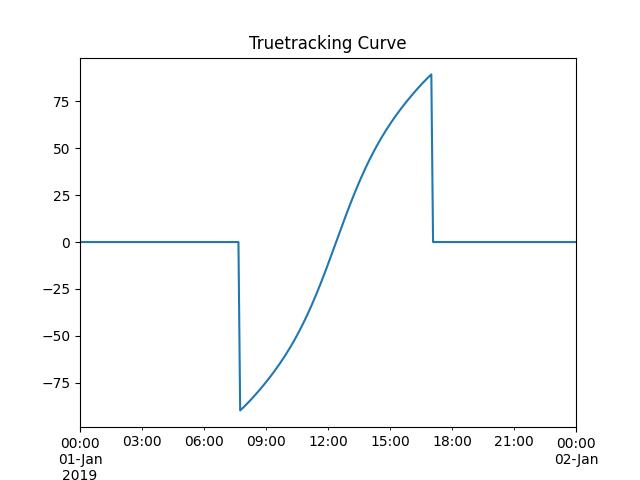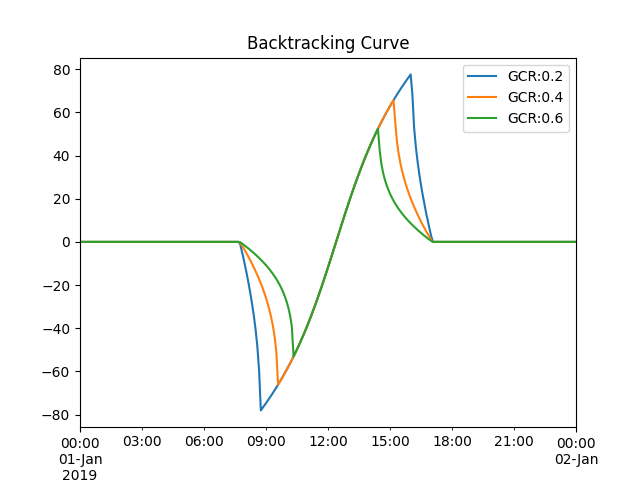# Single-axis tracking#

Examples of modeling tilt angles for single-axis tracker arrays.

This example shows basic usage of pvlib’s tracker position calculations with `pvlib.tracking.singleaxis()`. The examples shown here demonstrate how the tracker parameters affect the generated tilt angles.

Because tracker angle is based on where the sun is in the sky, calculating solar position is always the first step.

## True-tracking#

The basic tracking algorithm is called “true-tracking”. It orients the panels towards the sun as much as possible in order to maximize the cross section presented towards incoming beam irradiance.

```from pvlib import solarposition, tracking
import pandas as pd
import matplotlib.pyplot as plt

tz = 'US/Eastern'
lat, lon = 40, -80

times = pd.date_range('2019-01-01', '2019-01-02', freq='5min',
tz=tz)
solpos = solarposition.get_solarposition(times, lat, lon)

truetracking_angles = tracking.singleaxis(
apparent_zenith=solpos['apparent_zenith'],
apparent_azimuth=solpos['azimuth'],
axis_tilt=0,
axis_azimuth=180,
max_angle=90,
backtrack=False,  # for true-tracking
gcr=0.5)  # irrelevant for true-tracking

truetracking_position = truetracking_angles['tracker_theta'].fillna(0)
truetracking_position.plot(title='Truetracking Curve')

plt.show()
```## Backtracking#

Because truetracking yields steep tilt angle in morning and afternoon, it will cause row to row shading as the shadows from adjacent rows fall on each other. To prevent this, the trackers can rotate backwards when the sun is near the horizon – “backtracking”. The shading angle depends on row geometry, so the gcr parameter must be specified. The greater the gcr, the tighter the row spacing and the more aggressively the array must backtrack.

```fig, ax = plt.subplots()

for gcr in [0.2, 0.4, 0.6]:
backtracking_angles = tracking.singleaxis(
apparent_zenith=solpos['apparent_zenith'],
apparent_azimuth=solpos['azimuth'],
axis_tilt=0,
axis_azimuth=180,
max_angle=90,
backtrack=True,
gcr=gcr)

backtracking_position = backtracking_angles['tracker_theta'].fillna(0)
backtracking_position.plot(title='Backtracking Curve',
label=f'GCR:{gcr:0.01f}',
ax=ax)

plt.legend()
plt.show()
```Total running time of the script: (0 minutes 0.336 seconds)

Gallery generated by Sphinx-Gallery